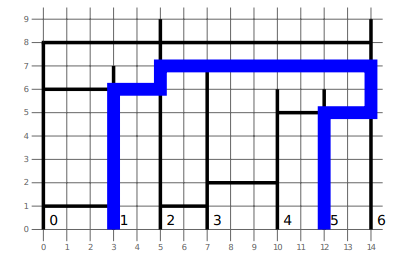## IOI '19 P6 - Sky Walking

View as PDF

Points: 40 (partial)
Time limit: 4.0s
Memory limit: 1G

Problem type
Allowed languages
C++

Kenan drew a plan of the buildings and skywalks along one side of the main avenue of Baku. There arebuildings numbered fromtoandskywalks numbered fromto. The plan is drawn on a two-dimensional plane, where the buildings and skywalks are vertical and horizontal segments respectively.

The bottom of buildingis located at pointand the building has height. Hence, it is a segment connecting the pointsand.

Skywalkhas endpoints at buildings numberedandand has a positive-coordinate. Hence, it is a segment connecting the pointsand.

A skywalk and a building intersect if they share a common point. Hence, a skywalk intersects two buildings at its two endpoints, and may also intersect other buildings in between.

Kenan would like to find the length of the shortest path from the bottom of buildingto the bottom of building, assuming that one can only walk along the buildings and skywalks, or determine that no such path exists. Note that it is not allowed to walk on the ground, i.e. along the horizontal line with-coordinate.

One can walk from a skywalk into a building or vice versa at any intersection. If the endpoints of two skywalks are at the same point, one can walk from one skywalk to the other.

#### Implementation details

You should implement the following procedure. It will be called by the grader once for each test case.

long long min_distance(std::vector<int> x, std::vector<int> h, std::vector<int> l, std::vector<int> r, std::vector<int> y, int s, int g)

•and: integer arrays of length•, and: integer arrays of length•and: two integers
• This procedure should return the length of the shortest path between the bottom of building and the bottom of building, if such path exists. Otherwise, it should return.

#### Examples

##### Example 1

Consider the following call:

min_distance([0, 3, 5, 7, 10, 12, 14],
[8, 7, 9, 7, 6, 6, 9],
[0, 0, 0, 2, 2, 3, 4],
[1, 2, 6, 3, 6, 4, 6],
[1, 6, 8, 1, 7, 2, 5],
1, 5)


The correct answer is.

The figure below corresponds to Example 1:##### Example 2
min_distance([0, 4, 5, 6, 9],
[6, 6, 6, 6, 6],
[3, 1, 0],
[4, 3, 2],
[1, 3, 6],
0, 4)


The correct answer is.

#### Constraints

•••for all•for all•for all••• No two skywalks have a common point, except maybe on their endpoints.

1. (points)2. (points) Each skywalk intersects at mostbuildings.
3. (points), and all buildings have the same height.
4. (points)5. (points) No additional constraints.

• line:• line:• line:• line:The sample grader prints a single line containing the return value of min_distance.